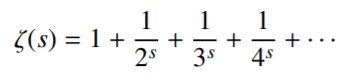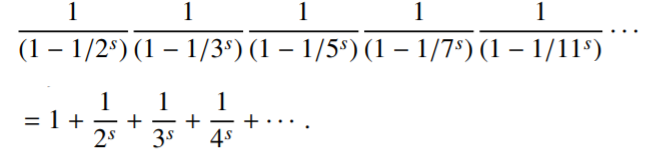# Riemann Zeta Function

Contents:

## What is the Riemann Zeta Function?

The Riemann Zeta Function is a generalized harmonic series, given by the formula:Where n and s are two real numbers.

• When n = 1, the zeta function is the same as the harmonic series. Like the harmonic series, it also diverges (fails to tend towards a certain number).
• For all other values of n, the series conditionally converges (tends toward a number).

The function is useful in several areas, including properties of prime numbers and definite integrals.

## History

Leonhard Euler was the first to study the function, but Bernhard Riemann was the first to study it extensively. Riemann was preoccupied with complex analysis and introduced the Zeta Function in during his study of primes; perhaps his most significant contribution is his consideration of the zeta-function as a type of analytic function (Patterson, 1995).

## The Riemann Hypothesis

Riemann discovered that the zeta function is more powerful when complex numbers are allowed. This led directly to the Riemann hypothesis.

Although many parts of the function have been investigated and proved, the Riemann hypothesis (simply stated as “The real part of every non-trivial zero of the Riemann zeta function is ½”) remains famously unproven.

The hypothesis starts with Euler’s formula:The factors on the left-hand side of the equation areWhere pn is the nth prime number.

The factor can be expanded as a geometric series:This is where it gets interesting: if you multiply all of the above series together, you get the reciprocal of every possible produce of primes (to the sth power), exactly once. But every natural number greater than or equal to 2 can only be expressed one way as a product of primes. This means that the expanded geometric series equals the right-hand side of Euler’s formula.

The exponent s > 1 was only placed in the formula to make sure the series converged. Riemann’s discovery made the function more powerful by adding the power of complex numbers, he essentially changed how the series works. While ζ(2), ζ(4), ζ(6), … were found by Euler, ζ(3), ζ(5), ζ(7) do not have any relationship to any standard constants. The question of proving that ζ(0) happens only when Re(s) = ½ (excluding trivial zeros) is the famous, unsolved Riemann hypothesis (Stillwell, 2010).

## Zeros of the Riemann Zeta Function

Zeros of a function are any input (i.e. any “x”) that results in the function equaling zero. For a basic function like y = 2(x), this is fairly easy to do, but it gets a little more complicated with the Riemann Zeta Function, mostly because it involves complex numbers. The even integers -2, -4, -6… are sometimes called the “trivial zeros” of the Riemann zeta function. However, other zeroes do exist and they are complex, “non-trivial” zeros. If you’re interested, Andrew Odlyzko has an extensive list, including the first 100 zeros (accurate to 1,000 decimal places). Here’s the first zero:

14.134725141734693790457251983562470270784257115699243175685567460149963429809256764949010393171561012779202971548797436766142691469882254582505363239447137780413381237205970549621955865860200555566725836010773700205410982661507542780517442591306254481978651072304938725629738321577420395215725674809332140034990468034346267314420920377385487141378317356396995365428113079680531491688529067820822980492643386667346233200787587617920056048680543568014444246510655975686659032286865105448594443206240727270320942745222130487487209241238514183514605427901524478338354254533440044879368067616973008190007313938549837362150130451672696838920039176285123212854220523969133425832275335164060169763527563758969537674920336127209259991730427075683087951184453489180086300826483125169112710682910523759617977431815170713545316775495153828937849036474709727019948485532209253574357909226125247736595518016975233461213977316005354125926747455725877801472609830808978600712532087509395997966660675378381214891908864977277554420656532052405

## Hurwitz Zeta Function

The “classical” Hurwitz zeta function (also called the generalized zeta function) is usually denoted by ζ (s, α) and is a generalization of the Riemann zeta function. The function, named after 19th century mathematician Adolf Hurwitz, can be defined for complex-numbered inputs s and a (with Re(a) > 0 and Re(s) > 1) as the series (Xu, 2018):The series is absolutely convergent for given values of a and a; It can also be extended to a meromorphic function for all s ≠ 1. When continued to the entire s-plane (the complex plane on which Laplace transforms are graphed), there is a simple pole at s = 1.

## Historical Note on the Riemann Zeta Function

The function can really only be understood by reading it in its historical context (including Riemann’s developments). According to legend, Hurwitz spent so much time pondering the Riemann hypothesis that his copy of Riemann’s collected works (obtained from his library after his death) automatically fell open to the paged where Riemann’s hypothesis was stated. For a great introduction, I recommend Harold Edward’s book Riemann’s Zeta Function; It’s an older text but an easy read compared to some of the more esoteric texts on the zeta function.

## Different Forms of the Hurwitz Zeta Function

This complex and interesting function has a plethora of different forms; It can be written as a sum of Dirichlet L-functions, as a series in terms of the gamma function, or in terms of the polygamma function (for positive integer inputs). When ζ(s, 1), the function becomes the Riemann zeta function.

Various extended forms of the function exist; A fairly simple version is the n-ple (“multiple”) Hurwitz zeta function, defined as (Srivastava & Choi, 2012):The Hurwitz Zeta Function function has a wide variety of applications in mathematics and quantum mechanics (Xu, 2018), including:

• Finding numerical prime density estimates (e.g. in the prime number theorem),
• Calculating the Casimir effect in quantum mechanics,
• Finding the pair production rate of Dirac particles.

## Dirichlet L-Function

A Dirichlet L-Function, a variation of the Riemann zeta function, is associated with a given Dirichlet character χ of a multiplicative group of integers modulo n. Each Dirichlet L-function has coefficients defined by different moduli (e.g. modulo 4, modulo 8,…). These L-functions are primarily used in analytic number theory and are part of the famous Riemann hypothesis.
It is given by (Li, 2019):As an example, the L-function of χ4, for Re(s) > 1, is (Conrad, 2018):Dirichlet introduced the Dirchlet character to prove that the number of primes in an arithmetic progression a, a + 2m, a + 3m is infinite when a and m contain zero common factors. In (relatively) simple terms, a Dirichlet character is periodic for some modulus and multiplicative.

Every Dirichlet L-function has an infinite number of nontrivial zeros (i.e. roots that are complex numbers).

## References

Baidoo, F. (2016). Dirichlet L-Functions and Dedekind η Functions. Retrieved December 2, 2020 from: https://math.uchicago.edu/~may/REU2016/REUPapers/Baidoo.pdf
Conrad, K. (2018). L-Functions and the Riemann Hypothesis. (Draft). Retrieved December 2, 2020 from: https://ctnt-summer.math.uconn.edu/wp-content/uploads/sites/1632/2018/05/ctnt2018-DirichletLfnGRH-Day1.pdf
Elizalde, E. et al. (1994). Zeta Regularization Techniques with Applications. World Scientific.
Li, W. (2019). Zeta and L-functions in Number Theory and Combinatorics. Conference Board of the Mathematical Sciences.
Patterson, S. (1995). An Introduction to the Theory of the Riemann Zeta-Function. Cambridge University Press.Srivastava, H. & Choi, J. (2012). Zeta and Q-Zeta Functions and Associated Series and Integrals. Elsevier Science.
Stillwell, J. (2010). Mathematics and Its History. 3rd Edition. Springer.
Xu, A. (2018). Approximating the Hurwitz Zeta Function. Retrieved July 20, 2020 from: https://amzn.to/32ByCAn

CITE THIS AS:
Stephanie Glen. "Riemann Zeta Function" From StatisticsHowTo.com: Elementary Statistics for the rest of us! https://www.statisticshowto.com/riemann-zeta-function/